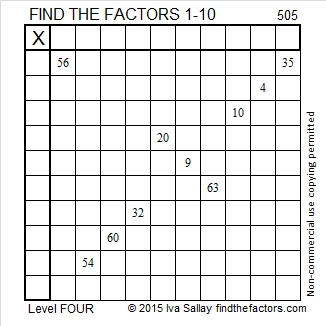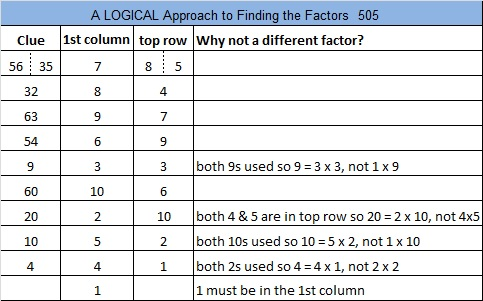# 505 and Level 4

I learned the coolest visual fact about the number 505 from OEIS.org:

505 = 10C5 + 10C0 + 10C5.Print the puzzles or type the solution on this excel file: 10 Factors 2015-05-25

—————————————————————————————————

• 505 is a composite number.
• Prime factorization: 505 = 5 x 101
• The exponents in the prime factorization are 1 and 1. Adding one to each and multiplying we get (1 + 1)(1 + 1) = 2 x 2 = 4. Therefore 505 has exactly 4 factors.
• Factors of 505: 1, 5, 101, 505
• Factor pairs: 505 = 1 x 505 or 5 x 101
• 505 has no square factors that allow its square root to be simplified. √505 ≈ 22.47220505—————————————————————————————————This site uses Akismet to reduce spam. Learn how your comment data is processed.